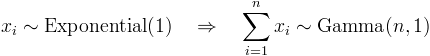Represents a Gamma distribution.Inheritance Hierarchy

Namespace:  Meta.Numerics.Statistics.Distributions
Assembly:  Meta.Numerics (in Meta.Numerics.dll) Version: 4.1.4Syntax
`public sealed class GammaDistribution : ContinuousDistribution`

The GammaDistribution type exposes the following members.Constructors
NameDescriptionGammaDistribution(Double)
Initializes a new instance of the standard Gamma distribution.GammaDistribution(Double, Double)
Initializes a new instance of a Gamma distribution with the given parameters.
TopProperties
NameDescriptionExcessKurtosis
Gets the excess kurtosis of the distribution.
(Overrides UnivariateDistributionExcessKurtosis.)Mean
Gets the mean of the distribution.
(Overrides UnivariateDistributionMean.)Median
Gets the median of the distribution.
(Inherited from ContinuousDistribution.)Scale
Gets the scale parameter for the distribution.Shape
Gets the shape parameter for the distribution.Skewness
Gets the skewness of the distribution.
(Overrides UnivariateDistributionSkewness.)StandardDeviation
Gets the standard deviation of the distribution.
(Inherited from UnivariateDistribution.)Support
Gets the interval over which the distribution is non-vanishing.
(Overrides ContinuousDistributionSupport.)Variance
Gets the variance of the distribution.
(Overrides UnivariateDistributionVariance.)
TopMethods
NameDescriptionCentralMoment
Computes a central moment of the distribution.
(Overrides ContinuousDistributionCentralMoment(Int32).)Cumulant
Computes a cumulant of the distribution.
(Overrides UnivariateDistributionCumulant(Int32).)Equals
Determines whether the specified object is equal to the current object.
(Inherited from Object.)ExpectationValue
Computes the expectation value of the given function.
(Inherited from ContinuousDistribution.)FitToSample
Computes the Gamma distribution that best fits the given sample.GetHashCode
Serves as the default hash function.
(Inherited from Object.)GetRandomValue
Generates a random variate.
(Overrides ContinuousDistributionGetRandomValue(Random).)GetRandomValues
Generates the given number of random variates.
(Inherited from ContinuousDistribution.)GetType
Gets the Type of the current instance.
(Inherited from Object.)Hazard
Computes the hazard function.
(Inherited from ContinuousDistribution.)InverseLeftProbability
Returns the point at which the cumulative distribution function attains a given value.
(Overrides ContinuousDistributionInverseLeftProbability(Double).)InverseRightProbability
Returns the point at which the right probability function attains the given value.
(Inherited from ContinuousDistribution.)LeftProbability
Returns the cumulative probability to the left of (below) the given point.
(Overrides ContinuousDistributionLeftProbability(Double).)ProbabilityDensity
Returns the probability density at the given point.
(Overrides ContinuousDistributionProbabilityDensity(Double).)RawMoment
Computes a raw moment of the distribution.
(Overrides ContinuousDistributionRawMoment(Int32).)RightProbability
Returns the cumulative probability to the right of (above) the given point.
(Overrides ContinuousDistributionRightProbability(Double).)ToString
Returns a string that represents the current object.
(Inherited from Object.)
TopRemarks

The sum of n exponentially distributed variates is a Gamma distributed variate.When the shape parameter is an integer, the Gamma distribution is also called the Erlang distribution. When the shape parameter is one, the Gamma distribution reduces to the exponential distribution.See Also